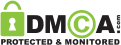In this level, you have to tell about 4+5=?. It is a very simple question but asked in a very confusing way. They have also given some more equations to distract and confuse the user. Most people got confused after watching the above equation and they try to solve the asked equation in the same manner as the above equation.

But the above equation is only to confuse you. Don’t get trapped.

You have to go straight here. Just focus of the asked equation that is 4+5=? and the answer of this is 4+5=9.

## All Level 1 to 225

Brain Out Finding Santa All levels 1 to 10

All Hints 1 to 225